# AP Chemistry : Le Chatelier's Principle

## Example Questions

← Previous 1

### Example Question #1 : Le Chatelier's Principle

Which of the following will cause an equilibrium shift in an exothermic reaction towards the products?

I. Decreasing the temperature

II. Evaporating the product

I and III only

I only

I and II only

III only

I, II, and III only

I and II only

Explanation:

I) Decreasing the temperature would take away heat from the system (a product), driving the reaction towards the products. II) Evaporating product would take a product away from the system, driving the reaction towards the products. III) Adding a catalyst only affects the rate of the reaction and does not effect equilibrium.

### Example Question #2 : Le Chatelier's Principle

According to Le Chatelier's principle, which of the following occurs when you compress a system containing at least one gas species?

shifts to favor the side with less moles of gas

not enough information to determine

shifts to favor the side with more moles of gas

remains at equilibrium

shifts to favor the side with less moles of gas

Explanation:

According to Le Chatelier's principle, when you compress a system, its volume decreases, so partial pressure of the all the gases in the system increases. The system will act to try to decrease the pressure by decreasing the moles of gas.

### Example Question #3 : Le Chatelier's Principle

If heat is added to an endothermic reaction, in which direction will the equilibrium shift according to Le Chatelier's principle?

to the left

to the right

cannot be determined

equilibrium does not shift

to the right

Explanation:

In an endothermic reaction, heat can be treated as a reactant. Thus, if you add more reactant (heat), the system will shift to get rid of the extra reactant and shift to the right to form more products.

### Example Question #4 : Le Chatelier's Principle

If heat is added to an exothermic reaction, in which direction will the equilibrium shift according to Le Chatelier's principle?

It cannot be determined.

Equilibrium does not shift.

It shifts to the right.

It shifts to the left.

It shifts to the left.

Explanation:

In an exothermic reaction, heat can be treated as a product. Thus, if you add more product (heat), the reaction will shift to the left to form more reactants.

### Example Question #5 : Le Chatelier's Principle

Which of the following stresses would lead the exothermic reaction below to shift to the right?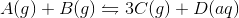Decreasing the volume

Increasing [A]

Increasing [C]

Increasing the temperature

Increasing [A]

Explanation:

By increasing the concentration of one of the reactants, the reaction will compensate by shifting to the right to increase production of products.

Increasing the concentration of one of the products (such as increasing [C]), however, would have the opposite effect. Increasing the temperature of an exothermic reaction would shift the reaction to the left, while increasing the temperature of an endothermic reaction would lead to a rightward shift. Finally, decreasing the volume leads to an increase in partial pressure of each gas, which the system compensates for by shifting to the side with fewer moles of gas. In this case, the right side has three moles of gas, while the left side has two; thus decreasing volume would shift equilibrium to the left.

### Example Question #6 : Le Chatelier's Principle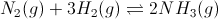Figure 1: Ammonia gas formation and equilibrium

What would most likely happen if a scientist decreased the volume of the container in which the reaction occurs?

More N2 would form

A violent explosion would occur

More H2 would form

More NH3 would form

Less NH3 would form

More NH3 would form

Explanation:

Le Chatelier's principle states that changes in pressure are attributable to changes in volume. If we increase the volume, the reaction will shift toward the side that has more moles of gas. If we decrease the volume, the reaction will shift toward the side that has less moles of gas. Since the product side has only two moles of gas, compared to the reactant side with four moles, the reaction would shift toward the product side, and more NH3 would form.

### Example Question #7 : Le Chatelier's Principle

Which of the following reactions will be favored when the pressure in a system is increased?

I.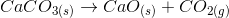II.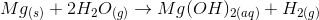III.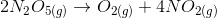I, II, and III

II and III only

I and II only

II only

I only

II only

Explanation:

With increased pressure, each reaction will favor the side with the least amount of moles of gas. In this problem we are looking for the reactions that favor the products in this scenario. I will favor reactants, II will favor products, III will favor reactants.

### Example Question #8 : Le Chatelier's Principle

Consider the following reaction system, which has a Keq of 1.35 * 104, taking place in a closed vessel at constant temperature.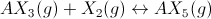AX5 is the main compound present

Increasing the pressure will produce more AX5

The rate of formation of AX5 equals the rate of formation of AX3 and X2

Increasing the volume will produce more AX5

Increasing the volume will produce more AX5

Explanation:

An increase in volume will result in a decrease in pressure at constant temperature. As a result, the equilibrium will shift toward the side with the greater total moles of gas, according to Le Chatelier's Principle. This will result in less AX5 being produced.

The Keq tells us that the reaction favors the products because it is greater than 1. The definition of equilibrium is that the rate of formation of products equals the rate of formation of reactants.

### Example Question #9 : Le Chatelier's Principle

What would happen to the Ksp if NH3 was added to an existing solution of Na2SO4?

It would increase.

It is impossible to determine.

It woud remain unchanged.

It would decrease.

It woud remain unchanged.

Explanation:

Ksp is dependent only on the species itself and the temperature of the solution. Adding another compound or stressing the system will not affect Ksp.

### Example Question #10 : Le Chatelier's Principle

Which of the following would occur if NH3 was added to an existing solution of Na2SO4?

It is impossible to determine

No effect

Na2SO4 will dissolve more